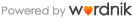# paragraph

paragraph

### 語源

• graph-, gram- : Gk.gramma = a letter(文字); Gk.graphein = to write(書く)

### 意味(英語)

• (noun) A distinct division of written or printed matter that begins on a new, usually indented line, consists of one or more sentences, and typically deals with a single thought or topic or quotes one speaker's continuous words.
• (noun) A mark ( ¶ ) used to indicate where a new paragraph should begin or to serve as a reference mark.
• (noun) A brief article, notice, or announcement, as in a newspaper.
• (verb-transitive) To divide or arrange into paragraphs.

### 例文

• (Beginning of paragraph following one on Unity in the paragraph) _The second of the essentials of the paragraph_, coherence, demands that ....## anagram

anagram

(文字の)つづり組替え ◇plumからlump、ana(＝逆に)＋gramma(＝文字)

#### 語源

• graph-, gram- : Gk.gramma = a letter(文字); Gk.graphein = to write(書く)

## gramophone

gramophone

(英・商標)蓄音機、(米)phonograph

#### 語源

• graph-, gram- : Gk.gramma = a letter(文字); Gk.graphein = to write(書く)

【広告】## telegraph

telegraph

#### 語源

• graph-, gram- : Gk.gramma = a letter(文字); Gk.graphein = to write(書く)

## autograph

autograph

#### 語源

• graph-, gram- : Gk.gramma = a letter(文字); Gk.graphein = to write(書く)

## graph

graph

グラフ、図表

#### 語源

• graph-, gram- : Gk.gramma = a letter(文字); Gk.graphein = to write(書く)

## telegraphy

telegraphy

#### 語源

• graph-, gram- : Gk.gramma = a letter(文字); Gk.graphein = to write(書く)

## autobiography

autobiography

#### 語源

• graph-, gram- : Gk.gramma = a letter(文字); Gk.graphein = to write(書く)

## graphic

graphic

グラフの、生き生きとした

#### 語源

• graph-, gram- : Gk.gramma = a letter(文字); Gk.graphein = to write(書く)

## biography

biography

#### 語源

• graph-, gram- : Gk.gramma = a letter(文字); Gk.graphein = to write(書く)

## graphite

graphite

(鉱)石墨、黒鉛、グラファイト(書くために鉛筆の芯に使用)

#### 語源

• graph-, gram- : Gk.gramma = a letter(文字); Gk.graphein = to write(書く)

【広告】## cablegram

cablegram

#### 語源

• graph-, gram- : Gk.gramma = a letter(文字); Gk.graphein = to write(書く)

## lithography

lithography

#### 語源

• graph-, gram- : Gk.gramma = a letter(文字); Gk.graphein = to write(書く)

## calligraphy

calligraphy

#### 語源

• graph-, gram- : Gk.gramma = a letter(文字); Gk.graphein = to write(書く)

## micrograph

micrograph

#### 語源

• graph-, gram- : Gk.gramma = a letter(文字); Gk.graphein = to write(書く)

## diagram

diagram

#### 語源

• graph-, gram- : Gk.gramma = a letter(文字); Gk.graphein = to write(書く)

## mimeograph

mimeograph

ガリ版、謄写版 ◇mineo(＝まねする)

#### 語源

• graph-, gram- : Gk.gramma = a letter(文字); Gk.graphein = to write(書く)

## epigram

epigram

(石の上に書いたもの、碑銘、簡潔な文)警句、風刺詩 ◇epi-(＝upon)

#### 語源

• graph-, gram- : Gk.gramma = a letter(文字); Gk.graphein = to write(書く)

## phonograph

phonograph

#### 語源

• graph-, gram- : Gk.gramma = a letter(文字); Gk.graphein = to write(書く)

【広告】## epigrammatic

epigrammatic

#### 語源

• graph-, gram- : Gk.gramma = a letter(文字); Gk.graphein = to write(書く)

## photograph

photograph

#### 語源

• graph-, gram- : Gk.gramma = a letter(文字); Gk.graphein = to write(書く)

## epigraph

epigraph

(上に刻まれた語) → 碑文、短句

#### 語源

• graph-, gram- : Gk.gramma = a letter(文字); Gk.graphein = to write(書く)

## photographer

photographer

#### 語源

• graph-, gram- : Gk.gramma = a letter(文字); Gk.graphein = to write(書く)

## geography

geography

#### 語源

• graph-, gram- : Gk.gramma = a letter(文字); Gk.graphein = to write(書く)

## photography

photography

#### 語源

• graph-, gram- : Gk.gramma = a letter(文字); Gk.graphein = to write(書く)

## glamorous

glamorous

(性的に)魅力的な

#### 語源

• graph-, gram- : Gk.gramma = a letter(文字); Gk.graphein = to write(書く)

## program

program

プログラム、計画(皆の前に示される書き物)

#### 語源

• graph-, gram- : Gk.gramma = a letter(文字); Gk.graphein = to write(書く)

【広告】## glamour/glamor

glamour/glamor

#### 語源

• graph-, gram- : Gk.gramma = a letter(文字); Gk.graphein = to write(書く)

## stenograph

stenograph

#### 語源

• graph-, gram- : Gk.gramma = a letter(文字); Gk.graphein = to write(書く)

## grammar

grammar

(書き方)文法(中世の)秘術

#### 語源

• graph-, gram- : Gk.gramma = a letter(文字); Gk.graphein = to write(書く)

## stenographer

stenographer

#### 語源

• graph-, gram- : Gk.gramma = a letter(文字); Gk.graphein = to write(書く)

## grammatical

grammatical

#### 語源

• graph-, gram- : Gk.gramma = a letter(文字); Gk.graphein = to write(書く)

## stenography

stenography

#### 語源

• graph-, gram- : Gk.gramma = a letter(文字); Gk.graphein = to write(書く)

## grammarian

grammarian

#### 語源

• graph-, gram- : Gk.gramma = a letter(文字); Gk.graphein = to write(書く)

## telegram

telegram

(遠くから書き送る)電報 ◇tele(＝遠く)

#### 語源

• graph-, gram- : Gk.gramma = a letter(文字); Gk.graphein = to write(書く)

【広告】